SSC BOARD PAPERS IMPORTANT TOPICS COVERED FOR BOARD EXAM 2024

### Practice Set 1.3 Linear Equations In Two Variables Class 10th Mathematics Part 1 MHB Solution

Practice Set 1.3

Fill in the blanks with correct number | ll 3&2 4&5 | = 3 x square - square x 4 =…

Find the values of following determinants. (1) | ll -1&7 2&4 | (2) | cc 5&3 -7&0 | (3)…

3x - 4y = 10; 4x + 3y = 5 Solve the following simultaneous equations using Cramer’s…

4x + 3y - 4 = 0; 6x = 8 - 5y Solve the following simultaneous equations using Cramer’s…

x + 2y = -1; 2x - 3y = 12 Solve the following simultaneous equations using Cramer’s…

6x - 4y = -12; 8x - 3y = -2 Solve the following simultaneous equations using Cramer’s…

4m + 6n = 54; 3m + 2n = 28 Solve the following simultaneous equations using Cramer’s…

2x+3y = 2 x - y/2 = 1/2 Solve the following simultaneous equations using Cramer’s…

###### Practice Set 1.3

Question 1.

Fill in the blanks with correct number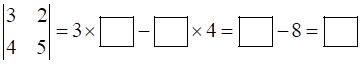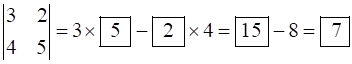Question 2.

Find the values of following determinants.

(1)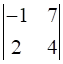(2)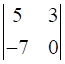(3)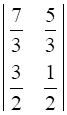we know, determinant of a 2 × 2 matrix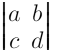(1) (–1× 4) – (7 × 2) = –4 – 14 = –18

(2) (5× 0)– (3× –7)= 0 –(–21) = 21

(3)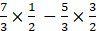=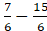Question 3.

Solve the following simultaneous equations using Cramer’s rule.

3x – 4y = 10; 4x + 3y = 5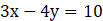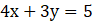D=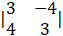=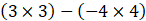=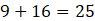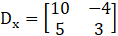=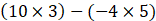=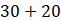= 50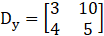=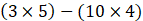=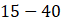= –25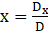=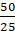= 2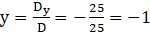∴ (x,y) = (2, –1) is the solution

Question 4.

Solve the following simultaneous equations using Cramer’s rule.

4x + 3y – 4 = 0; 6x = 8 – 5y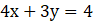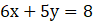D =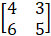=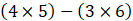= 20–18 =2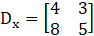=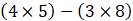= 20–24 =–4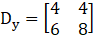=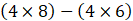= 32–24 = 8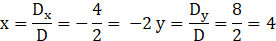∴(x,y)= (–2, 4) is the solution.

Question 5.

Solve the following simultaneous equations using Cramer’s rule.

x + 2y = –1; 2x – 3y = 12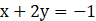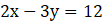D =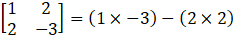= –3–4 = –7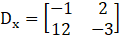=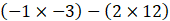= 3–24= –21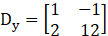=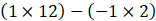=12+2 = 14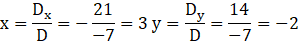∴ (x,y) = (3, –2) is solution.

Question 6.

Solve the following simultaneous equations using Cramer’s rule.

6x – 4y = –12; 8x – 3y = –2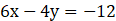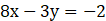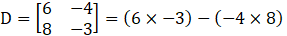= –18 + 32 = 14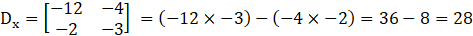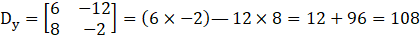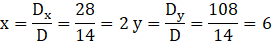∴ (x,y) = (2, 6) is solution.

Question 7.

Solve the following simultaneous equations using Cramer’s rule.

4m + 6n = 54; 3m + 2n = 28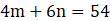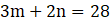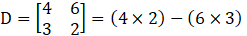= 8–18 =10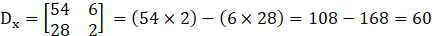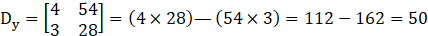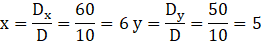∴ (x,y) = (6, 5) is solution.

Question 8.

Solve the following simultaneous equations using Cramer’s rule.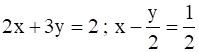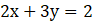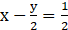⇒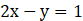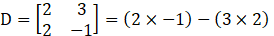= –2–6 = –8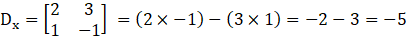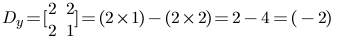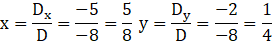∴ (x,y) =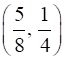is solution.